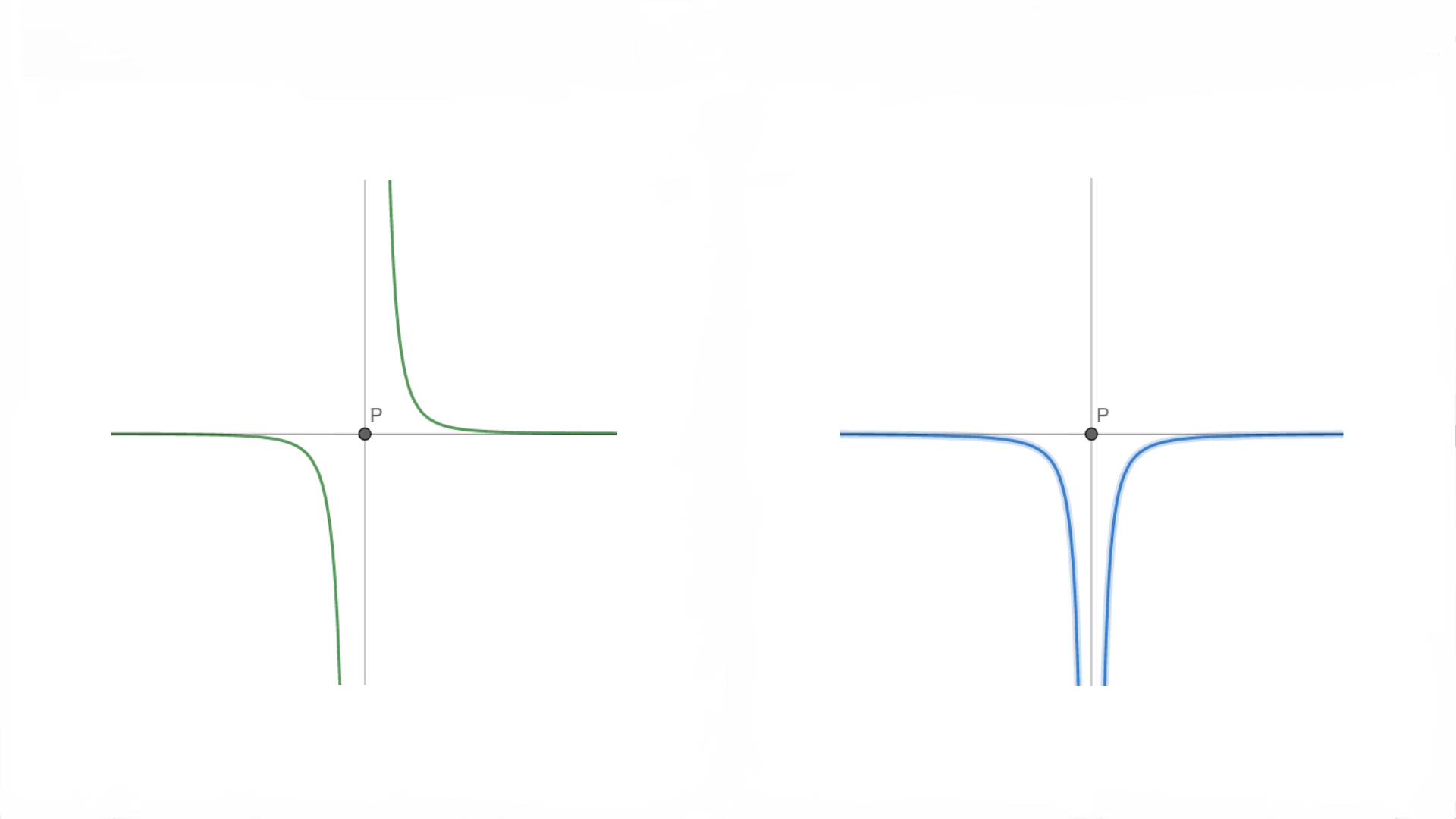# Fundamental Theorem of Analysis for discontinous functions

Last week I discovered a very neat property of improper Integrals. There are some functions where it is possible to calculate the area under the curve with the Fundamental Therorem of Analysis even if the function is discontinuous .

Let's say you have a function $f\left( x \right)$ and you want to calculate $\int _{ a }^{ b }{ f\left( x \right) dx }$. But unfortunately there is a pole point at ${ x }_{ p }$ with $b>{ x }_{ p }>a$.Now I will prove that: If ${ x }_{ p }$ is the only point on $\left[ a;b \right]$ where $f$ is discontinuos and there exists a point $P\left( { x }_{ p };{ y }_{ p } \right)$ to which $f$ is point symmetric, then it is possible to calculate the integral with the Fundamental Therorem of Analysis.

Because it is an improper integral, you devide the ingral into two: $\int _{ a }^{ b }{ f\left( x \right) dx } =\lim _{ z\rightarrow { 0 }^{ + } }{ \left( \int _{ a }^{ { x }_{ p }-z }{ f\left( x \right) dx } +\int _{ { x }_{ p }+z }^{ b }{ f\left( x \right) dx } \right) }$ Because ${ x }_{ p }$ is the only point of discontinuity of $f$, $f$ is continous on $\left[ a;{ x }_{ p }-z \right]$ and on $\left[ { x }_{ p }+z;b \right]$ and therefore you can calculate this with the Fundamental Therorem of Analysis, if $F$ is the antiderivative of $f$: \begin{aligned} \int _{ a }^{ b }{ f\left( x \right) dx } &= \lim _{ z\rightarrow { 0 }^{ + } }{ \left( F\left( { x }_{ p }-z \right) -F\left( a \right) +F\left( b \right) -F\left( { x }_{ p }+z \right) \right) } \\ \int _{ a }^{ b }{ f\left( x \right) dx } &= F\left( b \right) -F\left( a \right) +\lim _{ z\rightarrow { 0 }^{ + } }{ \left( F\left( { x }_{ p }-z \right) -F\left( { x }_{ p }+z \right) \right) } \end{aligned} If ${ x }_{ p }$ is a pole point of $f$ then it is a pole point of $F$ as well. $f$ is point symmetric to $P\left( { x }_{ p };{ y }_{ p } \right)$ and because $f$ is a measure of the slope of $F$ (because $\frac { d }{ dx } F\left( x \right) =f\left( x \right)$), $F$ must be axially symmetrical to $x={ x }_{ p }$.an example point symmetric function $f$ in green and a possible axial symmetrical antiderivative of $f$ in blue

And Therefore: $F\left( { x }_{ p }-z \right) =F\left( { x }_{ p }+z \right) \\ F\left( { x }_{ p }-z \right) -F\left( { x }_{ p }+z \right) =0$

$\int _{ a }^{ b }{ f\left( x \right) dx } =F\left( b \right) -F\left( a \right)$

The geometric interpretation of this relationship is, that the infinite areas on the left and right side of the pole cancel out each other because of the opposite sign.

It is important to mention, that the antiderivative must be defined for every $x\neq { x }_{ p }$ in $\left[ a;b \right]$, because otherwise it will not work.Note by CodeCrafter 1
1 year, 4 months ago

This discussion board is a place to discuss our Daily Challenges and the math and science related to those challenges. Explanations are more than just a solution — they should explain the steps and thinking strategies that you used to obtain the solution. Comments should further the discussion of math and science.

When posting on Brilliant:

• Use the emojis to react to an explanation, whether you're congratulating a job well done , or just really confused .
• Ask specific questions about the challenge or the steps in somebody's explanation. Well-posed questions can add a lot to the discussion, but posting "I don't understand!" doesn't help anyone.
• Try to contribute something new to the discussion, whether it is an extension, generalization or other idea related to the challenge.

MarkdownAppears as
*italics* or _italics_ italics
**bold** or __bold__ bold
- bulleted- list
• bulleted
• list
1. numbered2. list
1. numbered
2. list
Note: you must add a full line of space before and after lists for them to show up correctly
paragraph 1paragraph 2

paragraph 1

paragraph 2

[example link](https://brilliant.org)example link
> This is a quote
This is a quote
    # I indented these lines
# 4 spaces, and now they show
# up as a code block.

print "hello world"
# I indented these lines
# 4 spaces, and now they show
# up as a code block.

print "hello world"
MathAppears as
Remember to wrap math in $$ ... $$ or $ ... $ to ensure proper formatting.
2 \times 3 $2 \times 3$
2^{34} $2^{34}$
a_{i-1} $a_{i-1}$
\frac{2}{3} $\frac{2}{3}$
\sqrt{2} $\sqrt{2}$
\sum_{i=1}^3 $\sum_{i=1}^3$
\sin \theta $\sin \theta$
\boxed{123} $\boxed{123}$

Sort by:

Edit:

In this proof, I used the argument, that the antiderivative of $f$ must be axial symmetrical to $x={x}_{p}$. But this is not true for all functions.

For example if $f \left( x \right) = x^3 + 1$ then the antiderivative is $F \left( x \right) = \frac{1}{4} x^4 + x$. $f$ is point symmtric to $P \left( 0;1 \right)$ but $F$ is not axial symmetrical to $x=0$. But as we go closer and closer to the pole point, then we approach a "axial symmetry". Therefore near the pole point $F$ is axially symmetrical and hence the important limit in the proof above still converges to 0.

But unfortunately I cannot find a general argumentation, which guarantees that there are no counterexamples.

- 1 year, 4 months ago